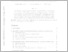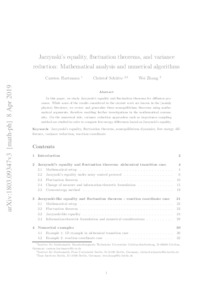Repository: Freie Universität Berlin, Math Department

# Jarzysnki equality, fluctuation theorems and variance reduction: Mathematical analysis and numerical algorithms

Hartmann, C. and Schütte, Ch. and Zhang, W. (2019) Jarzysnki equality, fluctuation theorems and variance reduction: Mathematical analysis and numerical algorithms. J. Stat. Phys., 175 (6). pp. 1214-1261. ISSN 0022-4715; ESSN: 1572-9613Preview

775kB

Official URL: https://dx.doi.org/10.1007/s10955-019-02286-4

## Abstract

In this paper, we study Jarzynski's equality and fluctuation theorems for diffusion processes. While some of the results considered in the current work are known in the (mainly physics) literature, we review and generalize these nonequilibrium theorems using mathematical arguments, therefore enabling further investigations in the mathematical community. On the numerical side, variance reduction approaches such as importance sampling method are studied in order to compute free energy differences based on Jarzynski's equality.

Item Type: Article SFB 1114 Preprint in arXiv:1803.09347 Mathematical and Computer Sciences > Mathematics > Applied Mathematics Department of Mathematics and Computer Science > Institute of Mathematics 2382 Silvia Hoemke 20 Nov 2019 11:00 20 Nov 2019 11:00

Repository Staff Only: item control page